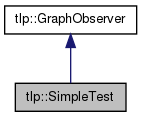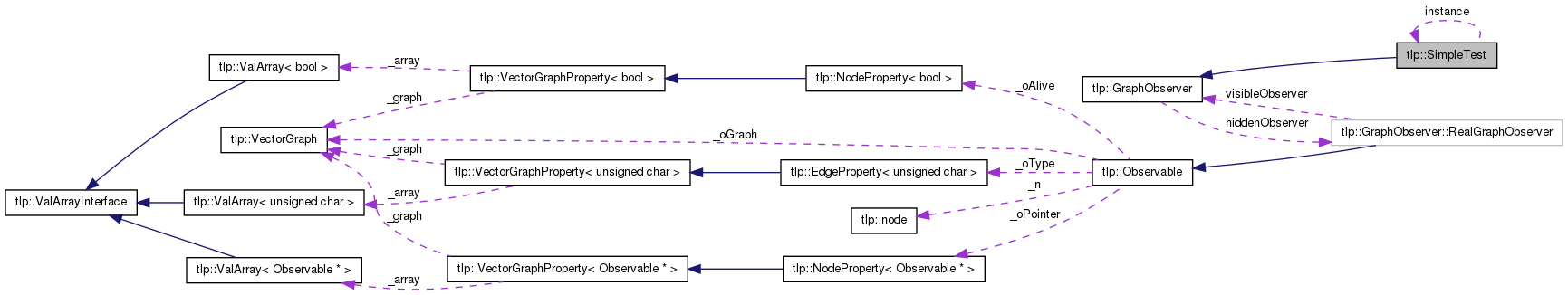Overview Modules Class Hierarchy Classes Members
tlp::SimpleTest Class Reference

`#include <SimpleTest.h>`

Inheritance diagram for tlp::SimpleTest:[legend]
Collaboration diagram for tlp::SimpleTest:[legend]

List of all members.

## Detailed Description

class for testing if the graph is simple (ie no self loops and no multiple or parallel edges)

## Member Function Documentation

 static bool tlp::SimpleTest::isSimple ( Graph * graph ) ` [static]`

Returns true if the graph is simple (i.e. it contains no self loops or parallel edges), false otherwise.

 static void tlp::SimpleTest::makeSimple ( Graph * graph, std::vector< edge > & removed ) ` [static]`

Makes the graph simple by removing self loops and parallel edges if any. The removed edges are stored in the removed vector.

 static bool tlp::SimpleTest::simpleTest ( Graph * graph, std::vector< edge > * multipleEdges = `0`, std::vector< edge > * loops = `0` ) ` [static]`

Performs simple test and stores found parallel edges in the multipleEdges vector and found self loops in the loops vector. Returns true if the graph is simple, false otherwise.

 Tulip Software by LaBRI Visualization Team    2001 - 2012